# Test: Hashing

## 10 Questions MCQ Test GATE Computer Science Engineering(CSE) 2023 Mock Test Series | Test: Hashing

Description
Attempt Test: Hashing | 10 questions in 30 minutes | Mock test for Computer Science Engineering (CSE) preparation | Free important questions MCQ to study GATE Computer Science Engineering(CSE) 2023 Mock Test Series for Computer Science Engineering (CSE) Exam | Download free PDF with solutions
QUESTION: 1

### A hash table of length 10 uses open addressing with hash function h(k)=k mod 10, and linear probing. After inserting 6 values into an empty hash table, the table is as shown below.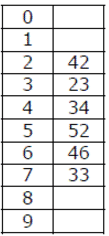Q. Which one of the following choices gives a possible order in which the key values could have been inserted in the table?

Solution:

The sequence (A) doesn’t create the hash table as the element 52 appears before 23 in this sequence.
The sequence (B) doesn’t create the hash table as the element 33 appears before 46 in this sequence.
The sequence (C) creates the hash table as 42, 23 and 34 appear before 52 and 33, and 46 appears before 33.
The sequence (D) doesn’t create the hash table as the element 33 appears before 23 in this sequence.

QUESTION: 2

###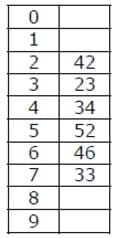How many different insertion sequences of the key values using the same hash function and linear probing will result in the hash table shown above?

Solution:

For the above hash table to occur, collision occurs for 52 and 33. That means, 42 and 23 must always appear before 52 and 33. Also note that 34 should always come before 52 and 33, else 52 and 33 may occupy 34's place. Therefore 42,23, and 34 should appear before 52 and 33. Hence 42, 23 and 34 can occur in 3! ways.
Now notice that 52 should come before, and finally 46 can appear at 5 different places.

Therefore, total number of different sequences = 3! x 5 = 30

QUESTION: 3

### The keys 12, 18, 13, 2, 3, 23, 5 and 15 are inserted into an initially empty hash table of length 10 using open addressing with hash function h(k) = k mod 10 and linear probing. What is the resultant hash table?

Solution:
• Open addressing, or closed hashing, is a method of collision resolution in hash tables. With this method a hash collision is resolved by probing, or searching through alternate locations in the array (the probe sequence) until either the target record is found, or an unused array slot is found, which indicates that there is no such key in the table.
• Well known probe sequences include: linear probing in which the interval between probes is fixed--often at 1. quadratic probing in which the interval between probes increases linearly (hence, the indices are described by a quadratic function). double hashing in which the interval between probes is fixed for each record but is computed by another hash function.
QUESTION: 4

Consider a hash table of size seven, with starting index zero, and a hash function (3x + 4)mod7. Assuming the hash table is initially empty, which of the following is the contents of the table when the sequence 1, 3, 8, 10 is inserted into the table using closed hashing? Note that ‘_’ denotes an empty location in the table.

Solution:

Let us put values 1, 3, 8, 10 in the hash of size 7. Initially, hash table is empty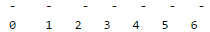The value of function (3x + 4)mod 7 for 1 is 0, so let us put the value at 0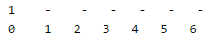The value of function (3x + 4)mod 7 for 3 is 6, so let us put the value at 6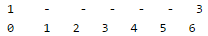The value of function (3x + 4)mod 7 for 8 is 0, but 0 is already occupied, let us put the value(8) at next available space(1)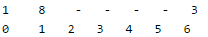The value of function (3x + 4)mod 7 for 10 is 6, but 6 is already occupied, let us put the value(10) at next available space(2)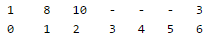QUESTION: 5

Given the following input (4322, 1334, 1471, 9679, 1989, 6171, 6173, 4199) and the hash function x mod 10, which of the following statements are true?
i. 9679, 1989, 4199 hash to the same value.
ii. 1471, 6171 has to the same value.
iii. All elements hash to the same value.
iv. Each element hashes to a different value.

Solution:

Hash function given is mod(10).
9679, 1989 and 4199 all these give same hash value i.e 9.
1471 and 6171 give hash value 1.

QUESTION: 6

Consider a hash table with 100 slots. Collisions are resolved using chaining. Assuming simple uniform hashing, what is the probability that the first 3 slots are unfilled after the first 3 insertions?

Solution:

Simple Uniform hashing function is a hypothetical hashing function that evenly distributes items into the slots of a hash table. Moreover, each item to be hashed has an equal probability of being placed into a slot, regardless of the other elements already placed.

► Probability that the first 3 slots are unfilled after the first 3 insertions =(probability that first item doesn't go in any of the first 3 slots)*
(probability that second item doesn't go in any of the first 3 slots)*
(probability that third item doesn't go in any of the first 3 slots)

= (97/100) * (97/100) * (97/100)

QUESTION: 7

Which one of the following hash functions on integers will distribute keys most uniformly over 10 buckets numbered 0 to 9 for i ranging from 0 to 2020?

Solution:

Since mod 10 is used, the last digit matters. If you do cube all numbers from 0 to 9, you get following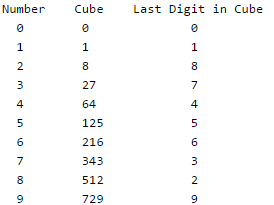Therefore all numbers from 0 to 2020 are equally divided in 10 buckets. If we make a table for square, we don't get equal distribution. In the following table. 1, 4, 6 and 9 are repeated, so these buckets would have more entries and buckets 2, 3, 7 and 8 would be empty.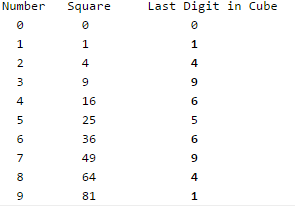QUESTION: 8

Given a hash table T with 25 slots that stores 2000 elements, the load factor α for T is __________

Solution:

load factor = (no. of elements) / (no. of table slots) = 2000/25 = 80

QUESTION: 9

Which of the following statement(s) is TRUE?

1. A hash function takes a message of arbitrary length and generates a fixed length code.
2. A hash function takes a message of fixed length and generates a code of variable length.
3. A hash function may give the same hash value for distinct messages.
Solution:

Hash function is defined as any function that can be used to map data of arbitrary size of data to a fixed size data.. The values returned by a hash function are called hash values, hash codes, digests, or simply hashes  : Statement 1 is correct Yes, it is possible that a Hash Function maps a value to a same location in the memmory that's why collision occurs and we have different technique to handle  this problem : Statement 3 is coorect. eg : we have hash function, h(x) = x mod 3 Acc to Statement 1, no matter what the value of 'x' is h(x) results in a fixed mapping location. Acc. to Statement 3, h(x) can result in same mapping mapping location for different value of 'x' e.g. if x = 4 or x = 7 , h(x) = 1 in both the cases, although collision occurs.

QUESTION: 10

Consider a hash function that distributes keys uniformly. The hash table size is 20. After hashing of how many keys will the probability that any new key hashed collides with an existing one exceed 0.5.

Solution:

For each entry probability of collision is 1/20 {as possible total spaces = 20, and an entry will go into only 1 place}
Say after inserting x values probability becomes ½
(1/20).x = ½
X = 10Use Code STAYHOME200 and get INR 200 additional OFF Use Coupon Code